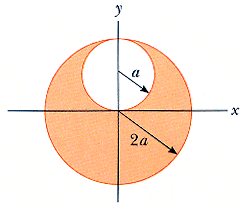# Gauss's Law Sphere Problem

A sphere of radius 2a is made of nonconducting material that has a uniform volume charge density . (Assume that the material does not affect the electric field.) A spherical cavity of radius a is now removed from the sphere, as shown in Figure P19.62. Show that the electric field within the cavity is uniform and is given by Ex = 0 and Ey = a/30. (Hint: The field within the cavity is the superposition of the field due to the original uncut sphere, plus the field due to a sphere the size of the cavity with a uniform negative charge density -.)I really don't have any idea how to solve this one. I have a vague idea about ratios of volume to charge. I know it must involve something with that because of the measurement of charge density, but some specifics would really be appreciated.

Shooting Star
Homework Helper
Have you done similar problems in finding the gravity at a certain depth inside a mine or some such stuff? It's a very common problem. If you don't have any idea, it becomes difficult for anybody to solve it wholly for you.

The fact you must know is that the field inside a very thin hollow uniform shell with uniform surface charge density is zero.

Yes, but the electric field in the cavity isn't zero. That's what initially confused me.

Shooting Star
Homework Helper
That only happens in a perfect conductor, irrespective of the shape of the cavity. This is different.

EDIT: Temporarily withdrawn rest of post, to think of easier solution.

Last edited:
Shooting Star
Homework Helper
First tell me what is the field at the surface of a uniformly charged sphere of total charge Q?

And the field inside a thick hollow spherical shell with uniform volume charge density?

I'm not sure why you'd want the hollow shell case...

The way I'd do it is to use Gauss' law to work out the vector E for a uniformly charged sphere (it is a very simple expression)
Then do the superposition of the two spheres at a given point r, remembering to allow for change of coordinates.

Shooting Star
Homework Helper
I'm not sure why you'd want the hollow shell case...

To use spherical symmetry and then use Gauss law. Could you use Gauss law to find the field inside a uniformly charged cube?

The way I'd do it is to use Gauss' law to work out the vector E for a uniformly charged sphere (it is a very simple expression)
Then do the superposition of the two spheres at a given point r, remembering to allow for change of coordinates.

That will be the correct way. However, the intention of the HW forum is not to solve it for the OP but to nudge him in the right direction, and give a big helping hand if need be over the difficult math parts. I am sure there are scores of people in PF who will readily solve the whole problem for him/her.

In this case, if the OP can't answer the simple questions that I've asked, then he/she is not ready for this problem.